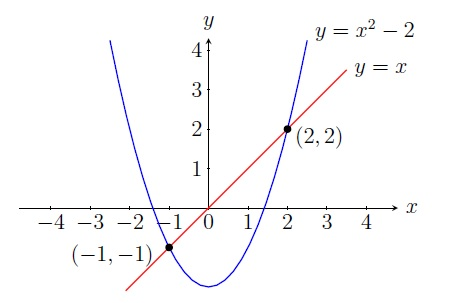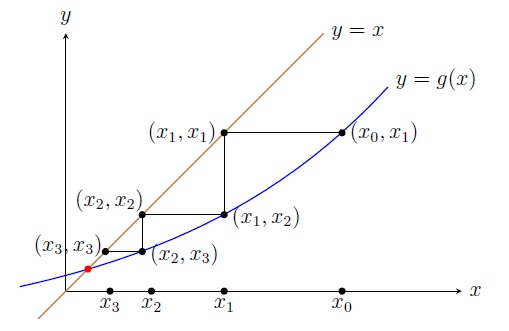Numerical Analysis Home

## Fixed Point Iteration

A number $$p$$ is a fixed point of a function $$g$$ if $$g(p)=p$$.

Example. If $$g(x)=x^2-2$$, then solving $$g(x)=x^2-2=x$$ we get $$x=-1,2$$. We can easily check $$g(-1)=-1$$ and $$g(2)=2$$. Thus $$-1$$ and $$2$$ are fixed points of $$g$$. Note that fixed points of $$g$$ are the $$x$$-value of the points of intersection of the curve $$y=g(x)$$ and the line $$y=x$$.The following shows the equivalence of root finding and finding fixed points.

Observation. $$p$$ is a fixed point of a function $$g$$ if and only if $$p$$ is a root of $$f(x)=x-g(x)$$.

If $$p$$ is a fixed point of a function $$g$$, then $$g(p)=p$$ and consequently $$f(p)=p-g(p)=0$$. The converse follows similarly. Note that there are multiple choices for $$f$$ such as $$f(x)=e^x(x-g(x))$$, $$f(x)=-1+e^{x-g(x)}$$ etc.

The following theorem gives us sufficient conditions for existence and uniqueness of a fixed point:

Theorem.(Fixed Point Theorem) Let $$g$$ be a function on $$[a,b]$$.

1. (Existence) If $$g$$ is continuous and $$a\leq g(x)\leq b$$ for all $$x\in [a,b]$$, then $$g$$ has a fixed point in $$[a,b]$$.

2. (Uniqueness) Moreover, if $$|g'(x)|<1$$ for all $$x\in(a,b)$$, then $$g$$ has a unique fixed point in $$[a,b]$$.

Proof. Suppose $$g$$ is continuous and $$a\leq g(x)\leq b$$ for all $$x\in [a,b]$$. If $$g(a)=a$$ or $$g(b)=b$$, then $$a$$ or $$b$$ is a fixed point of $$g$$. Otherwise $$g(a) > a$$ and $$g(b)< b$$ because $$a\leq g(x)\leq b$$ for all $$x\in [a,b]$$.

Define a new function $$h$$ by $$h(x)=x-g(x)$$. Since $$g$$ is continuous, $$h$$ is also continuous. Also note that $$h(a)=a-g(a)<0$$ and $$h(b)=b-g(b)>0$$. By the IVT, $$h(c)=0$$ for some $$c\in (a,b)$$. Now $$h(c)=c-g(c)=0\implies g(c)=c$$, i.e., $$c$$ is a fixed point of $$g$$.

Suppose $$|g'(x)|<1$$ for all $$x\in(a,b)$$. To show uniqueness of $$c$$, suppose $$d$$ is another fixed point of $$g$$ in $$[a,b]$$. WLOG let $$d>c$$. Applying the MVT on $$g$$ on $$[c,d]$$, we find $$t\in (c,d)$$ such that $g(d)-g(c)=g'(t)(d-c).$ Since $$|g'(x)|<1$$ for all $$x\in(a,b)$$, $g(d)-g(c)=g'(t)(d-c)<(d-c).$ Since $$g(c)=c$$ and $$g(d)=d$$, we have $$g(d)-g(c)=d-c$$ which contradicts that $$g(d)-g(c)<(d-c).$$ ◼

Suppose $$a\leq g(x)\leq b$$ for all $$x\in [a,b]$$ and $$|g'(x)|\leq k<1$$ for all $$x\in(a,b)$$. For any initial approximation $$x_0$$ in $$[a,b]$$, the fixed point iteration constructs a sequence $$\{x_n\}$$, where $\boxed{x_{n+1}=g(x_n),\;n=0,1,2,\ldots}$ to approximate the unique fixed point $$x^*$$ of $$g$$ in $$[a,b]$$. We will show $$\{x_n\}$$ converges to $$x^*$$.Example. This problem approximates the root $$x^*$$ of $$f(x)=e^x-x-2$$ on the interval $$[0,2]$$.

1. Find a function $$g$$ that has a unique fixed point which is the root $$x^*$$ of $$f(x)=e^x-x-2$$ on the interval $$[0,2]$$.

2. Do six iterations by the fixed point iteration to approximate the root $$x^*$$ of $$f(x)=e^x-x-2$$ on the interval $$[0,2]$$ using $$x_0=1$$.

Solution. 1. We need to find a function $$g$$ such that

• $$0\leq g(x)\leq 2$$ for all $$x\in [0,2]$$, and
• $$|g'(x)|<1$$ for all $$x\in(0,2)$$.

$$f(x)=e^x-x-2=0\implies x=e^x-2$$. But $$g(x)=e^x-2$$ does not satisfy (a) as $$g(0)=-2<0$$ and also (b) as $$g'(2)=e^2>1$$. Note that $f(x)=e^x-x-2=0\implies e^x=x+2 \implies x=\ln(x+2).$ Take $$g(x)=\ln(x+2)$$. Then $$g$$ is an increasing function and $$g(0)=\ln 2>0$$ and $$g(2)=\ln 4<2$$. Thus $$0\leq g(x)\leq 2$$ for all $$x\in [0,2]$$. Also $$|g'(x)|=\frac{1}{x+2}\leq \frac{1}{2}<1$$ for all $$x\in(0,2)$$. Thus $$g$$ has a unique fixed point in $$[0,2]$$ which is the root $$x^*$$ of $$f(x)=e^x-x-2$$ on the interval $$[0,2]$$. 2. Use $$g(x)=\ln(x+2)$$ and $$x_0=1$$. $\begin{array}{|l|l|l|} \hline n & x_{n-1} & x_n=g(x_{n-1})\\ \hline 1 & 1 & 1.0986\\ \hline 2 & 1.0986 & 1.1309\\ \hline 3 & 1.1309 & 1.1413\\ \hline 4 & 1.1413 & 1.1446\\ \hline 5 & 1.1446 & 1.1456\\ \hline 6 & 1.1457 & 1.1460\\ \hline \end{array}$ Since $$|x_6-x_5|=|1.1460-1.1456|=0.0004<0.001=10^{-3}$$, we can say that the root is $$x_6=1.1460$$ roughly correct to three decimal places (which is true indeed as $$x^*=1.146193$$).

The Maximum Error: Let $$\varepsilon_n$$ be the absolute error for the $$n$$th iteration $$x_n$$. Then \begin{align*} \varepsilon_n=|x^*-x_n|\leq & k^n \max\{x_0-a, b-x_0\} \text{ and}\\ \varepsilon_n=|x^*-x_n|\leq & \frac{k^n}{1-k}|x_1-x_0|. \end{align*}

Proof. Applying the MVT on $$g$$ on $$[x^*,x_{n-1}]$$, we find $$\xi_{n-1}\in (x^*,x_{n-1})$$ such that $$g(x^*)-g(x_{n-1})=g'(\xi_{n-1})(x^*-x_{n-1})$$. Then $|x^*-x_n|=|g(x^*)-g(x_{n-1})|=|g'(\xi_{n-1})||x^*-x_{n-1}|\leq k|x^*-x_{n-1}|.$ Continuing this process, we get $|x^*-x_n|\leq k|x^*-x_{n-1}|\leq k^2|x^*-x_{n-2}|\leq \cdots\leq k^n|x^*-x_{0}|.$ Since $$x^*,x_0\in (a,b)$$, we have $$|x^*-x_{0}|\leq \max\{x_0-a, b-x_0\}$$. Thus $$\varepsilon_n=|x^*-x_n|\leq k^n \max\{x_0-a, b-x_0\}$$.

Note that similarly applying the MVT on $$g$$ on $$[x_n,x_{n+1}]$$, we can show that $|x_{n+1}-x_n|\leq k^n|x_1-x_{0}|.$ For the other bound, let $$m>n\geq 0$$. Then \begin{align*} |x_m-x_n| &=|x_m-x_{m-1}+x_{m-1}-x_{m-2}+x_{m-2}-\cdots+x_{n+1}-x_n|\\ &\leq |x_m-x_{m-1}|+|x_{m-1}-x_{m-2}|+\cdots+|x_{n+1}-x_n|\\ &\leq k^{m-1}|x_1-x_{0}|+k^{m-2}|x_1-x_{0}|+\cdots+k^{n}|x_1-x_{0}|\\ &\leq k^n|x_1-x_{0}|(1+k+\cdots+k^{m-n-1})\\ &\leq k^n|x_1-x_{0}|\sum_{i=0}^{m-n-1}k^i \end{align*} Thus \begin{align*} \lim_{m\to \infty}|x_m-x_n| &\leq \lim_{m\to \infty}k^n|x_1-x_{0}|\sum_{i=0}^{m-n-1}k^i=k^n|x_1-x_{0}|\sum_{i=0}^{\infty}k^i\\ \implies |x^*-x_n| & \leq k^n|x_1-x_{0}|\sum_{i=0}^{\infty}k^i=\frac{k^n}{1-k}|x_1-x_0|\;\; (\text{as } 0< k <1). \; ◼\\ \end{align*}

Convergence: The sequence $$\{x_n\}$$ constructed by the fixed point iteration converges to the unique fixed point $$x^*$$ irrespective of choice of the initial approximation $$x_0$$ because $\lim_{n\to \infty}|x^*-x_n|\leq \lim_{n\to \infty}k^n \max\{x_0-a, b-x_0\}=0 \;(\text{as }0< k <1) \implies \lim_{n\to \infty}|x^*-x_n|=0.$ It converges really fast when $$k$$ is close to $$0$$.

Example. Find the number of iteration by the fixed point iteration that guarantees to approximate the root $$x^*$$ of $$f(x)=e^x-x-2$$ on $$[0,2]$$ using $$x_0=1$$ with accuracy within $$10^{-3}$$.

Solution. Consider $$g(x)=\ln(x+2)$$ on $$[0,2]$$ where $$|g'(x)|\leq \frac{1}{2}=k<1$$ for all $$x\in(0,2)$$. \begin{align*} & \varepsilon_n=|x^*-x_n|\leq k^n \max\{x_0-a, b-x_0\}=\left(\frac{1}{2}\right)^n\cdot 1<10^{-3} \\ \implies & 10^3< 2^{n}\\ \implies & \ln 10^3 < \ln 2^{n} \\ \implies & 3 \ln 10< n\ln 2 \\ \implies & \frac{3\ln 10}{\ln 2}\approx 9.97< n \end{align*} Thus 10th iteration guarantees to achieve accuracy of the root within $$10^{-3}$$. But note that $$|x^*-x_5|=|1.1461-1.1457|=0.0004<10^{-3}$$. So Thus accuracy within $$10^{-3}$$ is reached at 5th iteration (way before 10th iteration). Also note that the other bound of $$\varepsilon_n=|x^*-x_n|\leq \frac{k^n}{1-k}|x_1-x_0|$$ gives $$10.94< n$$ which does not improve our answer.

Algorithm Fixed-point-Iteration
Input: $$g(x)=\ln(x+2)$$, interval $$[0,2]$$, an initial approximation $$x_0$$, tolerance $$10^{-3}$$,
the maximum number of iterations $$50$$
Output: an approximate fixed point of $$g$$ on $$[0,2]$$ within $$10^{-3}$$ or a message of failure
set $$x=x_0$$ and $$xold=x_0$$;
for $$i=1$$ to $$50$$
$$x=g(x)$$;
if $$|x-xold|<10^{-3}$$         $$\%$$ checking required accuracy
FoundSolution= true;         $$\%$$ done
break;         $$\%$$ leave for environment
end if
$$xold=x$$;         $$\%$$ update $$xold$$ for the next iteration
end for
if FoundSolution
return $$x$$
else
print 'the required accuracy is not reached in 50 iterations'
end if

Last edited